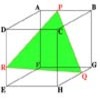#### You may also like### Cut Cube

Find the shape and symmetries of the two pieces of this cut cube.### Maximum Scattering

Your data is a set of positive numbers. What is the maximum value that the standard deviation can take?

# Walls

##### Age 16 to 18 Challenge Level:

Christopher from Farnborough 6th Form College and Tim from Royds School solved this problem using different methods so here are both their solutions. Christopher found the Cartesian equations of both planes and Tim's shorter method used vector and scalar products.

This is Christopher's solution: Let $P_1$ be a plane through the points $A(2,1,0), B(1,1,1)$ and $C(1,7,3)$ and $P_2$ be a plane through the points $A, B$ and $V(x,y,z)$. Find all the points $V(x,y,z)$ such that the two planes are perpendicular.

The general equation of a plane in 3 dimensional Cartesian space is: $$ax + by + cz + d = 0$$ This equation will be divided through by $a$, and slightly rearranged, to make the following process somewhat easier: $$x + py + qz = r$$ Where: $p = b/a,\ q = c/a$, and $r = -d/a$.

By substituting the $x, y$, and $z$ values from the points $A, B$, and $C$ into the general equation, it is possible to obtain the three equations below involving $p, q$, and $r$ that can be solved simultaneously to derive the formula of the plane $P_1$.

$$\begin{eqnarray} 2 + p &=& r \\ 1 + p + q &=& r\\ 1 + 7p + 3q &=& r \end{eqnarray}$$

Subtracting equation (2) from (1): $$1 - q = 0\ \rm {so}\ q = 1$$ Substituting this, and the relation in (1) into (3) gives:

$$\begin{eqnarray} 1 + 7p + 3 &=& 2 + p \\ 6p &=& -2 \\ p &=& -1/3 \end{eqnarray}$$

Finally, substituting this into (1) gives: $$2 - 1/3 = r\ \rm{so}\ r = 5/3.$$ Therefore, the equation of the plane $P_1$ is: $$x - 1/3y + z = 5/3.$$ Multiplying throughout by 3 gives: $$3x - y + 3z = 5.$$ Rearranging for y gives: $$y = 3x + 3z - 5$$ This implies that for every unit increase in the $x$ or $z$ direction, there is a corresponding increase of 3 in the $y$ direction. A normal plane would therefore have the property that for an increase of 3 in the $x$ or $z$ direction, there would be a corresponding increase of $-1$ in the $y$ direction. This implies that the vector $(3, -1, 3)$ describes a translation such that for any point on the plane $P_2$ (which is normal to $P_1$), the resulting point will also lie on $P_2$.

The translation is applied to point $A(2,1,0)$, giving the point $D(5,0,3)$. Using the points $A, B$, and $D$, which all lie on the plane $P_2$, the formula for $P_2$ can be determined:

$$\begin{eqnarray} 2 + p &=& r\\ 1 + p + q &=& r\\ 5 + 3q &=& r. \end{eqnarray}$$

From (4) - (5) $$1 - q = 0\ \rm {so}\ q = 1.$$ Substituting this into (6) gives: $$5 + 3 = r\ \rm {so}\ r = 8.$$ Substituting this into (2)gives: $$1 + p + 1 = 8\ \rm{so}\ p = 6.$$ Therefore the equation of the plane $P_2$ is: $$x + 6y + z = 8.$$ or: $$x + 6y + z - 8 = 0.$$ The point $V(x,y,z)$ lies on this plane; therefore, all the points $V(x,y,z)$ such that the two planes $P_1$ and $P_2$ are perpendicular are those that obey the equation above.

This is Tim's method:

Let $a \times b$ denote the cross product of $a$ and $b$. Let $v_1 = AC \times AB$ (which is perpendicular to $P_1$). Let $v_2 = AB \times AV$ (which is perpendicular to $P_2$).

As $P_1$ and $P_2$ are perpendicular, $v_1$ and $v_2$ are perpendicular as the angle between the planes is the same as that between their perpendiculars. Thus, the scalar product $v_1.v_2 = 0$.

$$\begin{eqnarray} AC &=& (1,7,3) - (2,1,0) &=& (-1,6,3)\\ AB &=& (1,1,1) - (2,1,0) &=& (-1,0,1)\\ AV &=& (x,y,z) - (2,1,0) &=& (x-2,y-1,z). \end{eqnarray}$$

$$v_1 = AC \times AB = (-1,6,3)\times(-1,0,1) = 6i - 2j + 6k$$ (which I evaluated using the determinant method)

$$v_2 = AB \times AV = (-1,0,1)\times(x-2,y-1,z) = (1-y)i +(x+z-2)j +(1-y)k$$ (again, using determinant method)

$$v_1.v_2 = 6 -6y -2x -2z + 4 + 6 -6y =0.$$ Therefore $2x + 12y + 2z = 16$ (rearranging) or, $x + 6y + z = 8$ (dividing by 2). This is the equation of the plane containing all $V(x,y,z)$ (the plane $P_2$)

Note: $(6,-2,6).(1,6,1) = 6 - 12 + 6 = 0$ i.e. the two planes are perpendicular (as required).

Also, $A$ and $B$ are in $P_2$ (as required) since $(2,1,0)$ and $(1,1,1)$ are solutions of the equation.